# 5G NR Downlink Carrier Waveform Generation

This example implements a 5G NR downlink carrier waveform generator using 5G Toolbox™.

### Introduction

This example shows how to parameterize and generate a 5G New Radio (NR) downlink waveform. The following channels and signals can be generated:

• PDSCH and its associated DM-RS and PT-RS

• PDCCH and its associated DM-RS

• PBCH and its associated DM-RS

• PSS and SSS

• CSI-RS

This example supports the parameterization and generation of multiple SCS specific carriers and multiple bandwidth parts (BWP). Multiple instances of the PDSCH and PDCCH channels can be generated over the different BWPs. Sets of CORESETs and search space monitoring opportunities can be configured for mapping the PDCCHs. Note that no precoding is applied to the physical channels and signals in this example.

### Waveform and Carrier Configuration

This section sets the SCS specific carrier bandwidths in resource blocks, the cell ID, and the length of the generated waveform in subframes. You can visualize the generated resource grids by setting the `DisplayGrids` field to 1. The channel bandwidth and frequency range parameters are used to display the associated minimum guardbands on a schematic diagram of the SCS carrier alignment.

```waveconfig = []; waveconfig.NCellID = 0; % Cell identity waveconfig.ChannelBandwidth = 40; % Channel bandwidth (MHz) waveconfig.FrequencyRange = 'FR1'; % 'FR1' or 'FR2' waveconfig.NumSubframes = 10; % Number of 1ms subframes in generated waveform (1,2,4,8 slots per 1ms subframe, depending on SCS) waveconfig.DisplayGrids = 1; % Display the resource grids after signal generation % Define a set of SCS specific carriers, using the maximum sizes for a % 40 MHz NR channel. See TS 38.101-1 for more information on defined % bandwidths and guardband requirements carriers(1).SubcarrierSpacing = 15; carriers(1).NRB = 216; carriers(1).RBStart = 0; carriers(2).SubcarrierSpacing = 30; carriers(2).NRB = 106; carriers(2).RBStart = 1; ```

### SS Burst

In this section you can set the parameters for the SS burst. The numerology of the SS burst can be different from other parts of the waveform. This is specified via the block pattern parameter as specified in TS 38.213 Section 4.1. A bitmap is used to specify which blocks are transmitted in a 5ms half-frame burst. The periodicity in milliseconds and the power of the burst can also be set here. Other SS burst parameters not shown here can also be set. For the full list see the help for `hSSBurst`.

```% SS burst configuration ssburst = []; ssburst.Enable = 1; % Enable SS Burst ssburst.BlockPattern = 'Case B'; % Case B (30kHz) subcarrier spacing ssburst.SSBTransmitted = [1 1 1 1]; % Bitmap indicating blocks transmitted in a 5ms half-frame burst ssburst.SSBPeriodicity = 20; % SS burst set periodicity in ms (5, 10, 20, 40, 80, 160) ssburst.FrequencySSB = 0*5000; % Frequency offset of SS burst (Hz), relative to waveform center (multiples of 5kHz) ssburst.Power = 0; % Power scaling in dB ```

### Bandwidth Parts

A BWP is formed by a set of contiguous resources sharing a numerology on a given carrier. This example supports the use of multiple BWPs using a structure array. Each entry in the array represents a BWP. For each BWP you can specify the subcarrier spacing (SCS), the cyclic prefix (CP) length and the bandwidth. The `SubcarrierSpacing` parameter maps the BWP to one of the SCS specific carriers defined earlier. The `RBOffset` parameter controls the location of the BWP in the carrier. This is expressed in terms of the BWP numerology. Different BWPs can overlap with each other.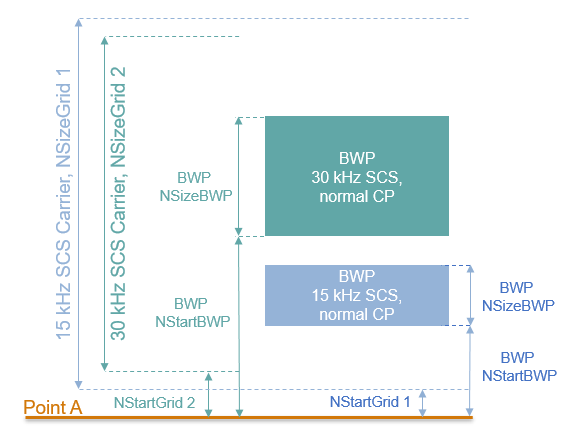```% Bandwidth parts configurations bwp = []; bwp(1).SubcarrierSpacing = 15; % BWP Subcarrier Spacing bwp(1).CyclicPrefix = 'Normal'; % BWP Cyclic prefix for 15 kHz bwp(1).NRB = 25; % Size of BWP bwp(1).RBOffset = 12; % Position of BWP in SCS carrier bwp(2).SubcarrierSpacing = 30; % BWP Subcarrier Spacing bwp(2).CyclicPrefix = 'Normal'; % BWP Cyclic prefix for 30 kHz bwp(2).NRB = 50; % Size of BWP bwp(2).RBOffset = 50; % Position of BWP in SCS carrier ```

### CORESET and Search Space Configuration

The parameters in this section specify the control resource set (CORESET) and the PDCCH search space configuration. The CORESET specifies the possible locations (in time and frequency) of the control channel for a given numerology. This example supports multiple CORESETs. The following parameters can be specified:

• Allocated OFDM symbols which specify the first symbol of each CORESET monitoring opportunity in a slot

• The allocated slots within a period

• Periodicity of the allocation. If this is set to empty it indicates no repetition

• CORESET duration in symbols, either 1, 2 or 3

• The allocated physical resource block (PRB) indices of the CORESET. Note that the CORESET frequency allocation is defined in blocks of 6 PRBs, aligned in common resource block (CRB) numbering, i.e. relative to point A. Each allocated PRB index input selects all 6 PRBs in the CRB aligned block that contains it.

• CCE-to-REG mapping which can be 'interleaved' or 'noninterleaved'

• Resource-element group (REG) bundle size (L), either (2,6) or (3,6), based on CORESET duration

• Interleaver size, either 2, 3, or 6

• Shift index, a scalar value in range 0...274

The figure below shows the meaning of some of the CORESET parameters.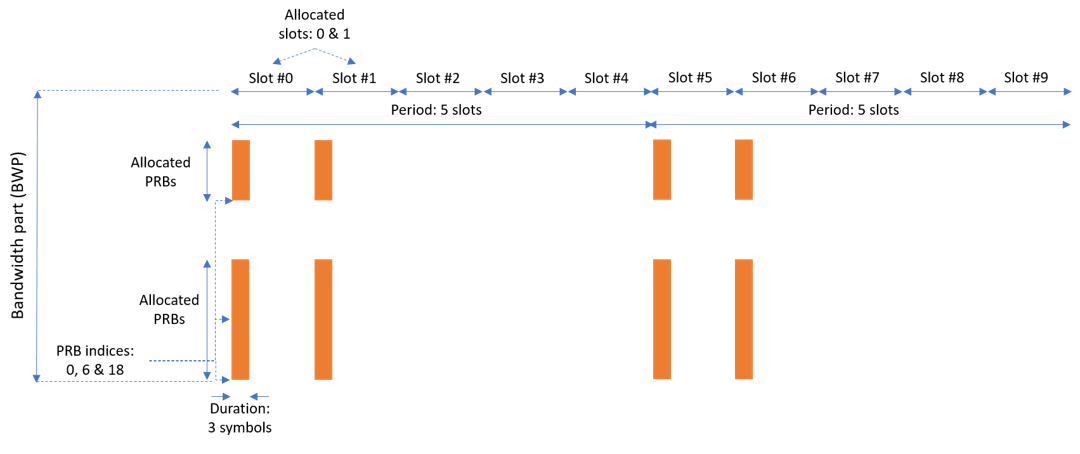```% CORESET/search space configurations coreset = []; coreset(1).AllocatedSymbols = [0,7]; % First symbol of each CORESET monitoring opportunity in a slot coreset(1).AllocatedSlots = [0,1]; % Allocated slots within a period coreset(1).AllocatedPeriod = 5; % Allocated slot period (empty implies no repetition) coreset(1).Duration = 3; % CORESET symbol duration (1,2,3) coreset(1).AllocatedPRB = 6*[0,1,3]; % 6 RB sized indices, relative to BWP (RRC - frequencyDomainResources) coreset(1).CCEREGMapping = 'noninterleaved'; % Mapping: 'interleaved' or 'noninterleaved' coreset(1).REGBundleSize = 3; % L (2,6) or (3,6) coreset(1).InterleaverSize = 2; % R (2,3,6) coreset(1).ShiftIndex = waveconfig.NCellID; % default to NCellID, else 0...274 ```

### PDCCH Instances Configuration

This section specifies the parameters for the set of PDCCH instances in the waveform. Each element in the structure array defines a PDCCH sequence instance. The following parameters can be set:

• Enable/disable the PDCCH sequence

• Specify the BWP carrying the PDCCH

• PDCCH instance power in dB

• Enable/disable DCI channel coding

• Allocated search spaces within the CORESET monitoring occasion sequence

• CORESET which carries the PDCCH instance

• Periodicity of the allocation. If this is set to empty it indicates no repetition

• Number of control channel elements (CCEs) in this PDCCH

• `NumCCE` and `StartCCE` specify the elements used for the transmission of this PDCCH

• RNTI

• Scrambling NID for this PDCCH and its associated DM-RS

• DM-RS power boosting

• DCI message data source. You can use one of the following standard PN sequences: 'PN9-ITU', 'PN9', 'PN11', 'PN15', 'PN23'. The seed for the generator can be specified using a cell array in the form `{'PN9',seed}`. If no seed is specified, the generator is initialized with all ones

```pdcch = []; pdcch(1).Enable = 1; % Enable PDCCH sequence pdcch(1).BWP = 1; % Bandwidth part pdcch(1).Power = 1.1; % Power scaling in dB pdcch(1).EnableCoding = 1; % Enable DCI coding pdcch(1).CORESET = 1; % Control resource set ID which carries this PDCCH pdcch(1).AllocatedSearchSpaces = [0,3]; % Index of allocated search spaces in the CORESET monitoring sequence pdcch(1).AllocatedPeriod = 4; % Allocation slot period (empty implies no repetition) pdcch(1).NumCCE = 8; % Number of CCE used by PDCCH pdcch(1).StartCCE = 0; % Starting CCE of PDCCH pdcch(1).RNTI = 0; % RNTI pdcch(1).NID = 1; % PDCCH and DM-RS scrambling NID pdcch(1).PowerDMRS = 0; % Additional power boosting in dB pdcch(1).DataBlkSize = 20; % DCI payload size pdcch(1).DataSource = 'PN9'; % DCI data source ```

### PDSCH Instances Configuration

This section specifies the set of PDSCH instances in the waveform. Each element in the structure array defines a PDSCH sequence instance. This example defines two PDSCH sequence instances.

General Parameters

Set these parameters for each PDSCH sequence instance:

• Enable or disable this PDSCH sequence

• Specify the BWP carrying the PDSCH. The PDSCH will use the SCS specified for this BWP

• Power scaling in dB

• Enable or disable DL-SCH transport channel coding

• Transport block data source. You can use one of the following standard PN sequences: 'PN9-ITU', 'PN9', 'PN11', 'PN15', 'PN23'. The seed for the generator can be specified using a cell array in the form `{'PN9', seed}`. If no seed is specified, the generator is initialized with all ones

• Target code rate used to calculate the transport block sizes

• Symbol modulation

• Number of layers

• Redundancy version (RV) sequence

• Enable or disable the interleaving of the virtual to physical resource block mapping. If this parameter is not specified, the direct, non-interleaved mapping is considered

• Bundle size for the interleaved map, specified by the higher layer parameter vrb-ToPRB-Interleaver. If this parameter is not specified, the bundle size is set to 2

```pdsch = []; pdsch(1).Enable = 1; % Enable PDSCH sequence pdsch(1).BWP = 1; % Bandwidth part pdsch(1).Power = 0; % Power scaling in dB pdsch(1).EnableCoding = 1; % Enable DL-SCH transport channel coding pdsch(1).DataSource = 'PN9'; % Channel data source pdsch(1).TargetCodeRate = 0.4785; % Code rate used to calculate transport block sizes pdsch(1).Xoh_PDSCH = 0; % Rate matching overhead pdsch(1).Modulation = 'QPSK'; % 'QPSK', '16QAM', '64QAM', '256QAM' pdsch(1).NLayers = 2; % Number of PDSCH layers pdsch(1).RVSequence = [0,2,3,1]; % RV sequence to be applied cyclically across the PDSCH allocation sequence pdsch(1).VRBToPRBInterleaving = 0; % Disable interleaved resource mapping pdsch(1).VRBBundleSize = 2; % vrb-ToPRB-Interleaver parameter ```

Allocation

The following diagram represents some of the parameters used in the PDSCH allocation.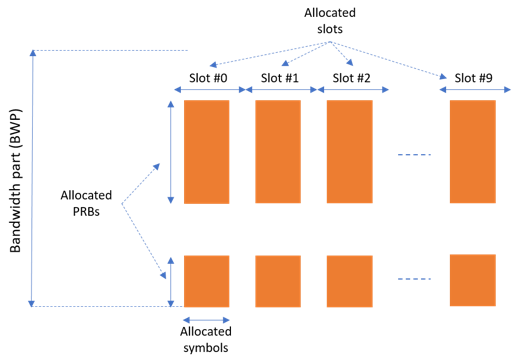You can set the following parameters to control the PDSCH allocation. Note that these parameters are relative to the BWP. The specified PDSCH allocation will avoid the locations used for the SS burst.

• Symbols in a slot allocated to each PDSCH instance

• Slots in a frame used for the sequence of PDSCH

• Period of the allocation in slots. If this is empty it indicates no repetition

• The allocated PRBs are relative to the BWP

• RNTI. This value is used to link the PDSCH to an instance of the PDCCH

• NID for scrambling the PDSCH bits

```pdsch(1).AllocatedSymbols = 2:10; % Range of symbols in a slot pdsch(1).AllocatedSlots = [0:9]; % Allocated slot indices for PDSCH sequence pdsch(1).AllocatedPeriod = 15; % Allocation period in slots (empty implies no repetition) pdsch(1).AllocatedPRB = [0:5, 10:20]; % PRB allocation pdsch(1).RNTI = 0; % RNTI pdsch(1).NID = 1; % Scrambling for data part ```

Note that the generator in this example does not check for inter-channel conflict. However, additional parameters can be specified for rate matching around other resources, if required

• The PDSCH can be rate matched around one or more CORESETs

• The PDSCH can be rate matched around other resource allocations

```pdsch(1).RateMatch(1).CORESET = ; % Rate matching pattern, defined by CORESET IDs pdsch(1).RateMatch(1).Pattern.AllocatedPRB = []; % Rate matching pattern, defined by set of 'bitmaps' pdsch(1).RateMatch(1).Pattern.AllocatedSymbols = []; pdsch(1).RateMatch(1).Pattern.AllocatedSlots = []; pdsch(1).RateMatch(1).Pattern.AllocatedPeriod = []; ```

PDSCH DM-RS Configuration

Set the DM-RS parameters

```% Antenna port and DM-RS configuration (TS 38.211 section 7.4.1.1) pdsch(1).PortSet = 0:pdsch(1).NLayers-1; % DM-RS antenna ports used pdsch(1).PDSCHMappingType = 'A'; % PDSCH mapping type ('A'(slot-wise),'B'(non slot-wise)) pdsch(1).DMRSTypeAPosition = 2; % Mapping type A only. First DM-RS symbol position (2,3) pdsch(1).DMRSLength = 1; % Number of front-loaded DM-RS symbols (1(single symbol),2(double symbol)) pdsch(1).DMRSAdditionalPosition = 0; % Additional DM-RS symbol positions (max range 0...3) pdsch(1).DMRSConfigurationType = 2; % DM-RS configuration type (1,2) pdsch(1).NumCDMGroupsWithoutData = 0; % CDM groups without data (max range 0...3) pdsch(1).NIDNSCID = 1; % Scrambling identity (0...65535) pdsch(1).NSCID = 0; % Scrambling initialization (0,1) pdsch(1).PowerDMRS = 0; % Additional power boosting in dB ```

PDSCH PT-RS Configuration

Set the PT-RS parameters

```% PT-RS configuration (TS 38.211 section 7.4.1.2) pdsch(1).EnablePTRS = 0; % Enable or disable the PT-RS (1 or 0) pdsch(1).PTRSTimeDensity = 1; % Time density (L_PT-RS) of PT-RS (1,2,4) pdsch(1).PTRSFrequencyDensity = 2; % Frequency density (K_PT-RS) of PT-RS (2,4) pdsch(1).PTRSREOffset = '00'; % PT-RS resource element offset ('00','01','10','11') pdsch(1).PTRSPortSet = 0; % PT-RS antenna ports must be a subset of DM-RS ports pdsch(1).PowerPTRS = 0; % Additional PT-RS power boosting in dB % When PT-RS is enabled, the DM-RS ports must be in range 0 to 3 for DM-RS % configuration type 1 and in range 0 to 5 for DM-RS configuration type 2. % Nominally the antenna port of PT-RS is the lowest DM-RS port number. ```

Specifying Multiple PDSCH Instances

A second PDSCH sequence instance is specified next using the second BWP.

```pdsch(2) = pdsch(1); pdsch(2).Enable = 1; pdsch(2).BWP = 2; % PDSCH mapped to 2nd BWP pdsch(2).AllocatedSymbols = 0:11; pdsch(2).AllocatedSlots = [2:4,6:20]; pdsch(2).AllocatedPRB = [25:30, 35:38]; % PRB allocation, relative to BWP ```

### CSI-RS

This section configures channel state information reference signals (CSI-RS) in the waveform. Each element in the structure array represents a set of CSI-RS resources associated with a BWP.

General Parameters

Set these parameters for a set of CSI-RS resources:

• Enable or disable this set of CSI-RS resources

• Specify the BWP carrying this set of CSI-RS resources. The CSI-RS resource(s) configuration will use the SCS specified for this BWP

• Specify the power scaling in dB. Providing a scalar defines the power scaling for a single CSI-RS resource or all configured CSI-RS resources. Providing a vector defines a separate power level for each of the CSI-RS resources.

```csirs(1).Enable = 0; csirs(1).BWP = 1; csirs(1).Power = 3; % in dB ```

CSI-RS configuration

You can configure the following parameters for one or more zero-power (ZP) or non-zero-power (NZP) CSI-RS resource configurations.

• Type of CSI-RS resource(s) ('nzp','zp')

• Row number corresponds to CSI-RS resource(s) as defined in TS 38.211 Table 7.4.1.5.3-1 (1...18)

• Frequency density of CSI-RS resource(s) ('one','three','dot5even','dot5odd')

• Subcarrier locations of CSI-RS resource(s) within a resource block (RB)

• Number of RBs allocated to CSI-RS resource(s) (1...275)

• Starting RB index of CSI-RS resource(s) allocation relative to the carrier resource grid (0...2169)

• OFDM symbol locations of CSI-RS resource(s) within a slot

• Allocated slots (0-based) of CSI-RS resource(s) within a period. This parameter can be a vector or a cell array of vectors. In the latter case, each cell corresponds to an individual CSI-RS resource. In case of a vector, the same set of slots is used for all CSI-RS resources

• Periodicity of CSI-RS resource(s) allocation in slots. If this is empty it indicates no repetition. This parameter can be a scalar or a cell array. In the latter case, each cell corresponds to an individual CSI-RS resource. In case of a scalar, the same period is used for all CSI-RS resources

• Scrambling identity corresponds to CSI-RS resource(s) for pseudo-random sequence generation (0...1023)

```csirs(1).CSIRSType = {'nzp','zp'}; csirs(1).RowNumber = [3 5]; csirs(1).Density = {'one','one'}; csirs(1).SubcarrierLocations = {6,4}; csirs(1).NumRB = 25; csirs(1).RBOffset = 12; csirs(1).SymbolLocations = {13,9}; csirs(1).AllocatedSlots = {[0 2],[2 3]}; csirs(1).AllocatedPeriod = {[],5}; csirs(1).NID = 5; ```

Specifying Multiple CSI-RS Instances

A set of CSI-RS resources associated with the second BWP.

```csirs(2).Enable = 0; csirs(2).BWP = 2; csirs(2).Power = 3; % in dB csirs(2).CSIRSType = {'nzp','nzp'}; csirs(2).RowNumber = [1 1]; csirs(2).Density = {'three','three'}; csirs(2).SubcarrierLocations = {0,0}; csirs(2).NumRB = 50; csirs(2).RBOffset = 50; csirs(2).SymbolLocations = {6,10}; csirs(2).AllocatedSlots = {[0,1],[0,1]}; csirs(2).AllocatedPeriod = {10,10}; csirs(2).NID = 0; ```

### Waveform Generation

This section collects all the parameters into the carrier configuration and generates the waveform.

```% Collect together channel oriented parameter sets into a single % configuration waveconfig.SSBurst = ssburst; waveconfig.Carriers = carriers; waveconfig.BWP = bwp; waveconfig.CORESET = coreset; waveconfig.PDCCH = pdcch; waveconfig.PDSCH = pdsch; waveconfig.CSIRS = csirs; % Generate complex baseband waveform [waveform,bwpset] = hNRDownlinkWaveformGenerator(waveconfig); ```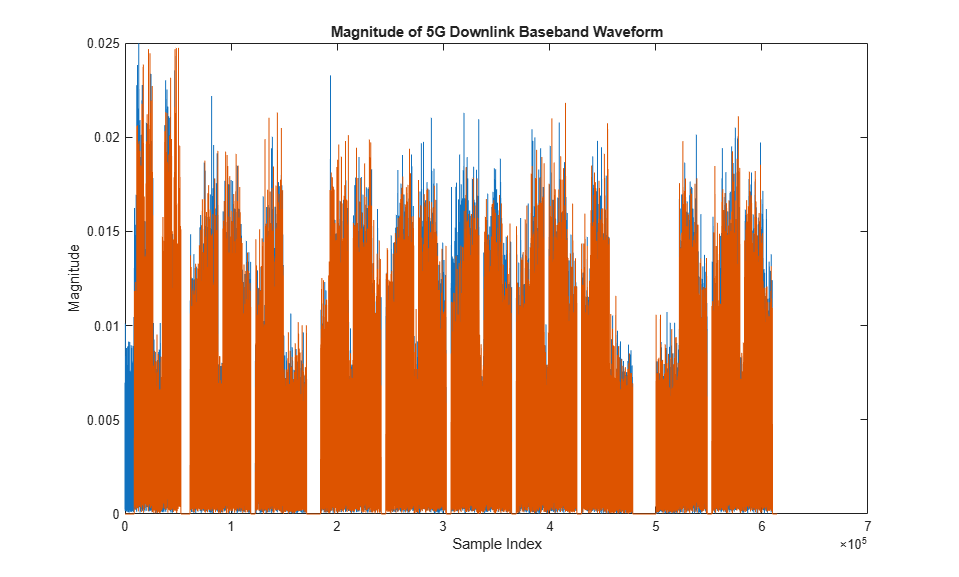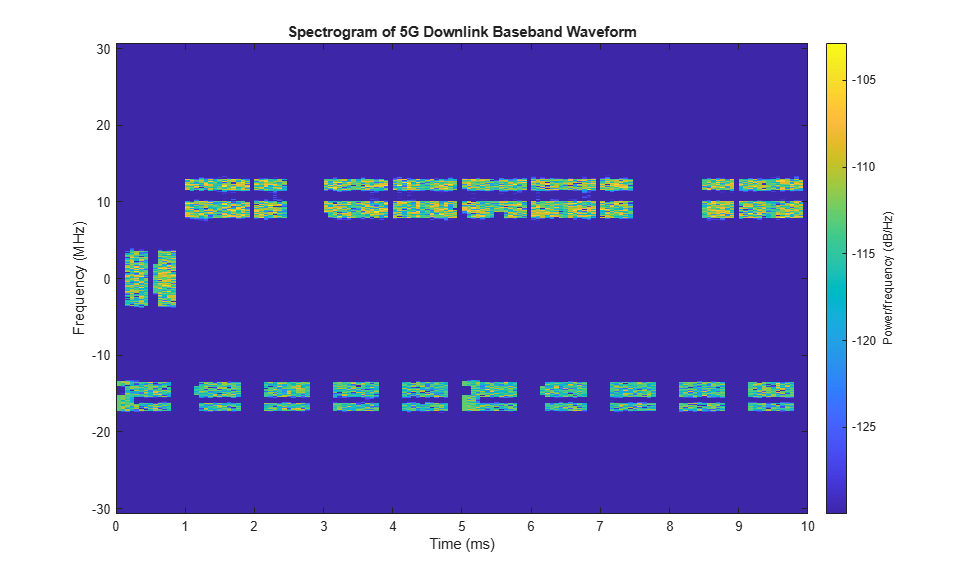The waveform generator also plots the resource grids for the bandwidth parts (this is controlled by the field `DisplayGrids` in the carrier configuration). The following plots are generated:

• The SCS specific carrier resource grids, along with the minimum guardbands, aligned relative to the overall channel bandwidth.

• Resource grids showing the location of the components (PDCCH, PDSCH, and CORESET) in each BWP. This does not plot the power of the signals, just their location in the grid.

• Generated waveform in the frequency domain for each BWP. This includes the PDCCH and PDSCH instances.

Note that none of these resource grids include the SS burst which is independent of the BWPs.

The waveform generator function returns the time domain waveform and a structure array `bwpset`, which contains the following fields:

• The resource grid corresponding to this BWP

• The resource grid of the overall bandwidth containing the channels and signals in this BWP

• An info structure with information corresponding to the BWP. The contents of this info structure for the first BWP are shown below.

```disp('Information associated to BWP 1:') disp(bwpset(1).Info) ```
```Information associated to BWP 1: SamplingRate: 61440000 Nfft: 4096 Windowing: 10 CyclicPrefixLengths: [1x14 double] SymbolLengths: [1x14 double] NSubcarriers: 2592 SubcarrierSpacing: 15 SymbolsPerSlot: 14 SlotsPerSubframe: 1 SymbolsPerSubframe: 14 SamplesPerSubframe: 61440 SubframePeriod: 1.0000e-03 Midpoints: [1x141 double] WindowOverlap: [10 10 10 10 10 10 10 10 10 10 10 10 10 10] k0: 0 ```

Note that the generated resource grid is a 3D matrix where the different planes represent the antenna ports. For the different physical channels and signals the lowest port is mapped to the first plane of the grid.